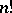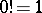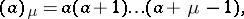Factorial

(diff) ← Older revision | Latest revision (diff) | Newer revision → (diff)

The function defined on the set of non-negative integers with value atequal to the product of the natural numbers from 1 to, that is, to; it is denoted by(by definition,). For largean approximate expression for the factorial is given by the Stirling formula. The factorial is equal to the number of permutations ofelements. The more general expressionis also called a factorial, whereis a complex number,is a natural number, and. See also Gamma-function.

Becauseequals the number of permutations ofelements, the factorial is extensively used in combinatorics, probability theory, mathematical statistics, etc. Cf. Combinatorial analysis; Combination; Binomial coefficients.